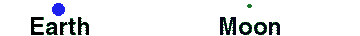Math & Science Home | Proficiency Tests | Mathematical Thinking in Physics | Aeronauts 2000

 CONTENTS Introduction Fermi's Piano Tuner Problem How Old is Old? If the Terrestrial Poles were to Melt... Sunlight Exerts Pressure Falling Eastward What if an Asteroid Hit the Earth Using a Jeep to Estimate the Energy in Gasoline How do Police Radars really work? How "Fast" is the Speed of Light? How Long is a Light Year? How Big is a Trillion? "Seeing" the Earth, Moon, and Sun to Scale Of Stars and Drops of Water If I Were to Build a Model of the Cosmos... A Number Trick Designing a High Altitude Balloon Pressure in the Vicinity of a Lunar Astronaut Space Suit due to Outgassing of Coolant Water Calendar Calculations Telling Time by the Stars - Sidereal Time Fields, an Heuristic Approach The Irrationality ofThe Irrationality ofThe Number (i)i Estimating the Temperature of a Flat Plate in Low Earth Orbit Proving that (p)1/n is Irrational when p is a Prime and n>1 The Transcendentality ofIdeal Gases under Constant Volume, Constant Pressure, Constant Temperature and Adiabatic Conditions Maxwell's Equations: The Vector and Scalar Potentials A Possible Scalar Term Describing Energy Density in the Gravitational Field A Proposed Relativistic, Thermodynamic Four-Vector Motivational Argument for the Expression-eix=cosx+isinx Another Motivational Argument for the Expression-eix=cosx+isinx Calculating the Energy from Sunlight over a 12 hour period Calculating the Energy from Sunlight over actual full day Perfect Numbers-A Case Study Gravitation Inside a Uniform Hollow Sphere Further note on Gravitation Inside a Uniform Hollow Sphere Pythagorean Triples Black Holes and Point Set Topology Additional Notes on Black Holes and Point Set Topology Field Equations and Equations of Motion (General Relativity) The observer in modern physics A Note on the Centrifugal and Coriolis Accelerations as Pseudo Accelerations - PDF File On Expansion of the Universe - PDF File

## "Seeing" the Earth, Moon, and Sun to Scale

The moon is about 1.3 light-seconds away (240,000 miles). Here is a scale picture of the Earth-moon system, with the earth (actual diameter: 8,000 miles) represented by a circle just a little bigger than 1/8 inch:(Remember this picture the next time you watch the movie Apollo 13!)

The sun is 8.3 light-minutes away (93,000,000 miles). A scale drawing is not as easily made here as it was for the Earth and moon above. If we chose the same 1/8 inch diameter circle to represent the earth, then we would require a circle bigger than one foot in diameter to represent the sun (actual diameter: 864,000 miles). The center of the sun circle would have to be placed about 121 feet from the center of the earth circle! You can begin to be impressed by the different sizes and separations that we encounter in space.

The terms light-seconds and light-minutes are used as units of distance along with light-years. These units may be understood as distances, or in another way. When we look up, we see the moon and sun, not as they are now, but as they were 1.3 seconds and 8.3 minutes ago, respectively. Hence, the concept of the space-time continuum: the further out we look into space, the farther back we see into time.